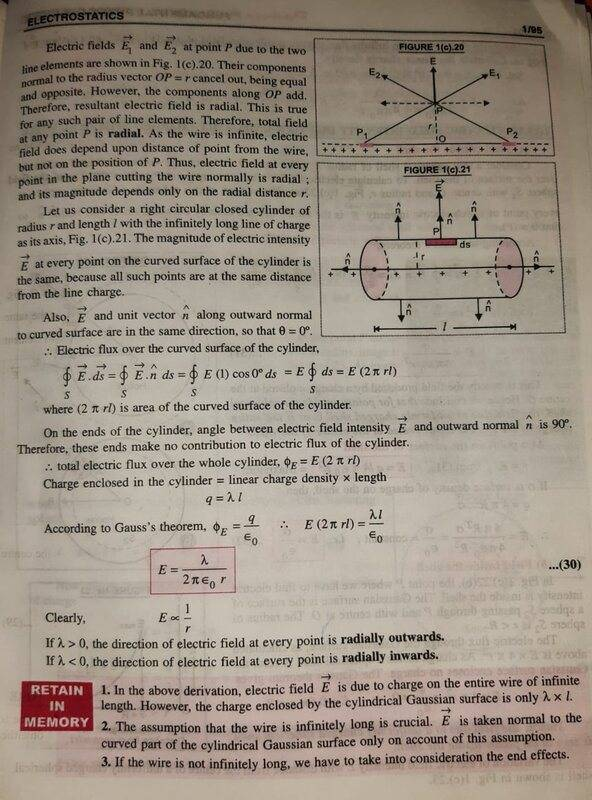# Electric Field Intensity due to an infinitely long straight uniformly charged wire

SHASHWAT PRATAP SINGThis the photo of my textbook, Here In the derivation of Electric Field Intensity due to an infinitely long straight uniformly charged wire -
In the figure 1(c).20 Vector E1 and vector E2 are electric fields at point P due to the two line elements as shown in the figure 1(c).20.
But, in this figure 1(c).20 two Electric field lines are shown intersecting PE1 and PE2, how can this be done as we know that Two electric field lines never intersect each other then how are they intersecting here...

If anyone wants to know what electric field lines are you can go here-
https://byjus.com/physics/electric-field-lines/

Last edited:

Homework Helper
Gold Member
The two angled lines that appear to be intersecting add together to give E that points straight up.

Homework Helper
Gold Member
2022 Award
If there are charges at ##P_1## and ##P_2##, then they both contribute to the electric field everywhere, including at point ##P##. Using Coulomb's law. Right?

SHASHWAT PRATAP SING
If there are charges at ##P_1## and ##P_2##, then they both contribute to the electric field everywhere, including at point ##P##. Using Coulomb's law. Right?
Yes.
But, the Electric Field Lines can never intersect each other, then why they are intersecting it here.

Homework Helper
Gold Member
2022 Award
Yes.
But, the Electric Field Lines can never intersect each other, then why they are intersecting it here.
You need to think about things more. "Electric field lines can never intersect". What does that mean?

SHASHWAT PRATAP SING
Homework Helper
Gold Member
2022 Award
SHASHWAT PRATAP SING
Perok, see the figure 1(c).20,
The Electric field lines PE1 and PE2 due to the two line elements are intersecting but how can these electric field lines intersect, as we know that electric field lines do not intersect each other.

Homework Helper
Gold Member
2022 Award
Perok, see the figure 1(c).20,
The Electric field lines PE1 and PE2 due to the two line elements are intersecting but how can these electric field lines intersect, as we know that electric field lines do not intersect each other.

Okay, I'll give you a mutiple choice:

a) Electric field lines can intersect.
b) Those lines in your book are not electric field lines.
c) The electric field lines from two different electric fields can intersect.

SHASHWAT PRATAP SING
Okay, I'll give you a mutiple choice:

a) Electric field lines can intersect.
b) Those lines in your book are not electric field lines.
c) The electric field lines from two different electric fields can intersect.
I guess maybe option (B)...
but if they are not electric field lines then what are they...

Homework Helper
Gold Member
2022 Award
I guess maybe option (B)...
but if they are not electric field lines then what are they...
We could go with that answer. Now that you don't have a fundamental objection to Coulomb's law, what happens when you apply it in this case?

•SHASHWAT PRATAP SING
Homework Helper
Gold Member
2022 Award
PS the first paragraph spells out what's happening. It's the vector addition of the electric fields associated with different charges.

SHASHWAT PRATAP SING
PS the first paragraph spells out what's happening. It's the vector addition of the electric fields associated with different charges.
Perok, can you please explain in detail I am not able to understand...

Staff Emeritus
I am not able to understand...

I'm not surprised, since you are answering him so quickly there is hardly enough time to type, let alone think.

Homework Helper
Gold Member
2022 Award
Perok, can you please explain in detail I am not able to understand...
It's 1) Coulomb's law; and 2) the vector addition of electric fields.

If that's a problem, then the material is too advanced and you need to take a step back and revise the basics.

SHASHWAT PRATAP SING
It's 1) Coulomb's law; and 2) the vector addition of electric fields.

If that's a problem, then the material is too advanced and you need to take a step back and revise the basics.
No, perok that's not the problem.
I just wanted a detailed explination.
But, Really Thanks for your help..

The Electric Field lines cannot intersect each othe but the Electric Field vector can intersect each other. That is what happening here...

Hassan latif
View attachment 280905
This the photo of my textbook, Here In the derivation of Electric Field Intensity due to an infinitely long straight uniformly charged wire -
In the figure 1(c).20 Vector E1 and vector E2 are electric fields at point P due to the two line elements as shown in the figure 1(c).20.
But, in this figure 1(c).20 two Electric field lines are shown intersecting PE1 and PE2, how can this be done as we know that Two electric field lines never intersect each other then how are they intersecting here...

If anyone wants to know what electric field lines are you can go here-
https://byjus.com/physics/electric-field-lines/